# What is Graphs Theory

Mathematics

In mathematics, graph theory is the study of graphs, which are mathematical structures used to model pairwise relations between objects. A graph in this context is made up of vertices (also called nodes or points) which are connected by edges (also called links or lines).

Computer Science

it is considered an abstract data type that is really good for representing connections or relations – unlike the tabular data structures of relational database systems, which are ironically very limited in expressing relations.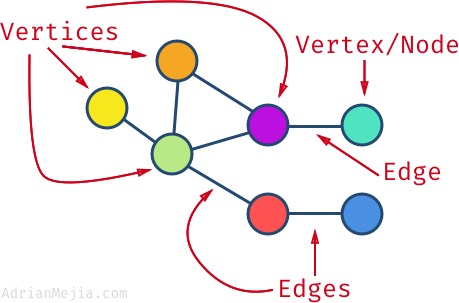## Graph Data Structure

A graph is a non-linear data structure consisting of nodes and edges. The nodes are sometimes also referred to as vertices and the edges are lines or arcs that connect any two nodes in the graph. More formally a Graph can be defined as, a Graph consists of a finite set of vertices(or nodes) and set of Edges which connect a pair of nodes.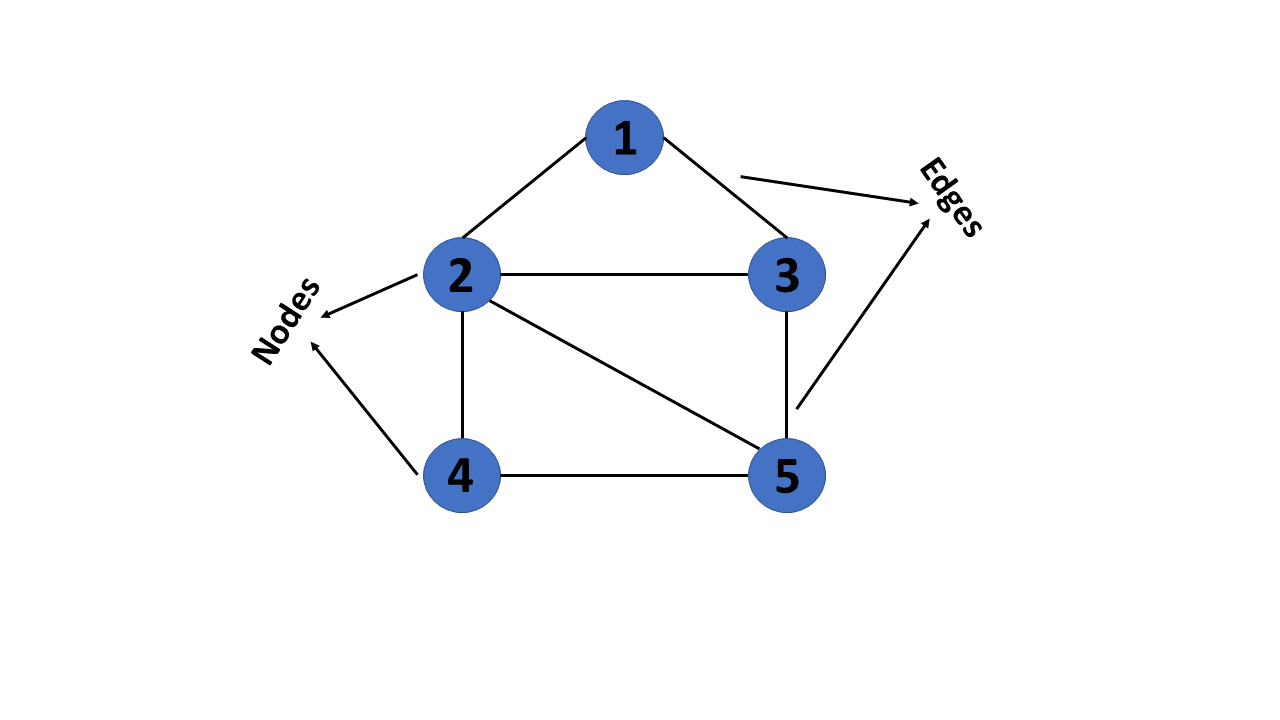In graph theory, a graph is a mathematical structure consisting of a set of objects, called vertices or nodes, and a set of connections, called edges, which link pairs of vertices. The notation:

$G = (V, E)$

is used to represent a graph, where $$G$$ is the graph, $$V$$ is the set of vertices, and $$\bigvee$$ is the set of edges.

The nodes of a graph can represent any objects, such as cities, people, web pages, or molecules, and the edges represent the relationships or connections between them.

import networkx as nx
import matplotlib.pyplot as plt

G = nx.Graph()
G.add_edges_from([('A', 'B'), ('A', 'C'), ('B', 'D'), ('B', 'E'), ('C', 'F'), ('C', 'G')])

plt.axis('off')
nx.draw_networkx(G,
pos=nx.spring_layout(G, seed=0),
node_size=600,
cmap='coolwarm',
font_size=14,
font_color='white'
)

/home/docs/checkouts/readthedocs.org/user_builds/ml-math/envs/latest/lib/python3.10/site-packages/networkx/drawing/nx_pylab.py:433: UserWarning: No data for colormapping provided via 'c'. Parameters 'cmap' will be ignored
node_collection = ax.scatter(## Graphs Terminology

The following are the most commonly used terms in graph theory with respect to graphs:

1. Vertex - A vertex, also called a “node”, is a fundamental part of a graph. In the context of graphs, a vertex is an object which may contain zero or more items called attributes.

2. Edge - An edge is a connection between two vertices. An edge may contain a weight/value/cost.

3. Path - A path is a sequence of edges connecting a sequence of vertices.

4. Cycle - A cycle is a path of edges that starts and ends on the same vertex.

5. Weighted Graph/Network - A weighted graph is a graph with numbers assigned to its edges. These numbers are called weights.

6. Unweighted Graph/Network - An unweighted graph is a graph in which all edges have equal weight.

7. Directed Graph/Network - A directed graph is a graph where all the edges are directed.

8. Undirected Graph/Network - An undirected graph is a graph where all the edges are not directed.

9. Adjacent Vertices - Two vertices in a graph are said to be adjacent if there is an edge connecting them.

## Types of Graphs

There are many types of graphs:

1. Directed Graphs

2. Undirected Graphs

3. Weighted Graph

4. Cyclic Graph

5. Acyclic Graph

6. Directed Acyclic Graph

Graphs have many properties, including the direction of travel for each relationship. The decision of which graph type you use usually depends on the use case.

There may not be an explicit direction from one node to another, such as with friendships in a social network, but in others, there might be clearly defined directions, such as flights and airports dataset.

### Directed Graphs

In a directed graph, all the edges are directed. That means, each edge is associated with a direction. For example, if there is an edge from node A to node B, then the edge is directed from A to B and not the other way around.

Directed graph, also called a digraph.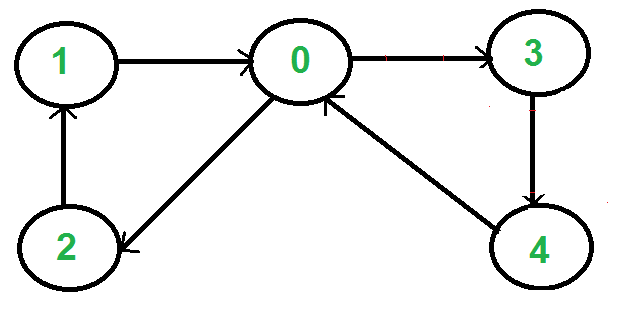DG = nx.DiGraph()
DG.add_edges_from([('A', 'B'), ('A', 'C'), ('B', 'D'),
('B', 'E'), ('C', 'F'), ('C', 'G')])
nx.draw_networkx(DG, pos=nx.spring_layout(DG, seed=0), node_size=600, cmap='coolwarm', font_size=14, font_color='white')### Undirected Graphs

In an undirected graph, all the edges are undirected. That means, each edge is associated with a direction. For example, if there is an edge from node A to node B, then the edge is directed from A to B and not the other way around.G = nx.Graph()
G.add_edges_from([('A', 'B'), ('A', 'C'), ('B', 'D'),
('B', 'E'), ('C', 'F'), ('C', 'G')])

nx.draw_networkx(G, pos=nx.spring_layout(G, seed=0), node_size=600, cmap='coolwarm', font_size=14, font_color='white')### Weighted Graph

In a weighted graph, each edge is assigned a weight or a cost. The weight can be positive, negative or zero. The weight of an edge is represented by a number. A graph G= (V, E) is called a labeled or weighted graph because each edge has a value or weight representing the cost of traversing that edge.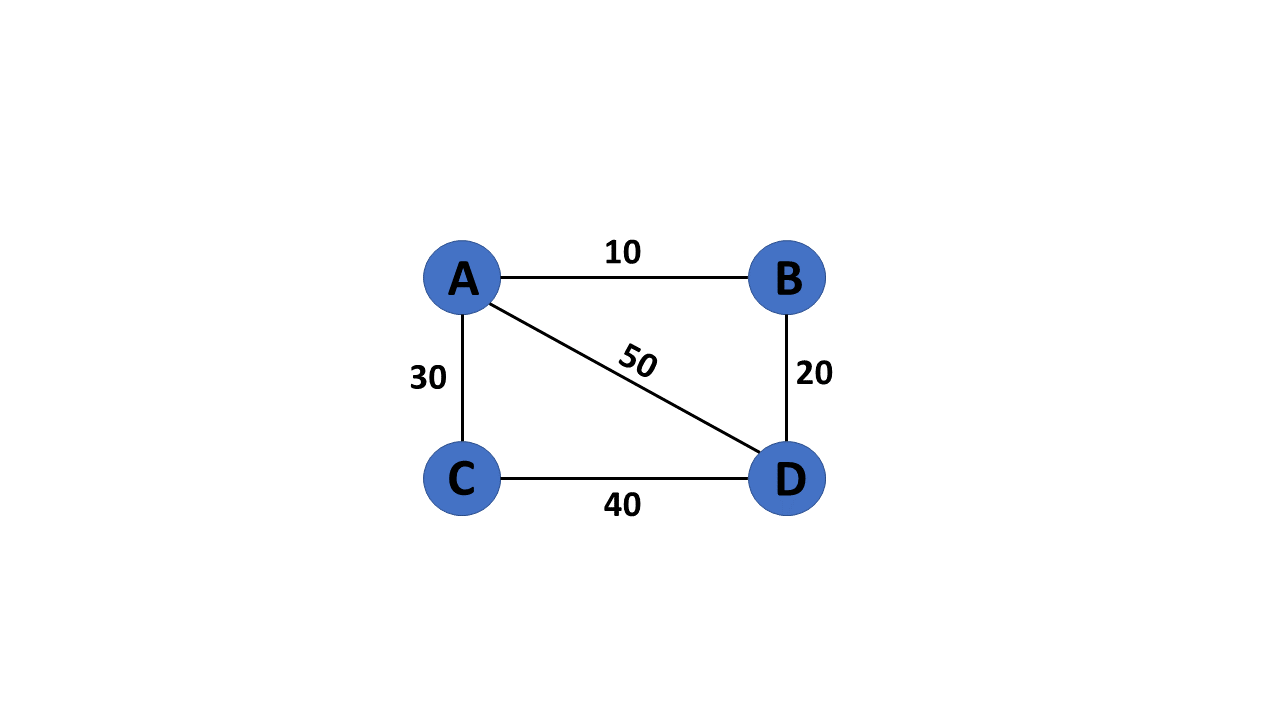WG = nx.Graph()
WG.add_edges_from([('A', 'B', {"weight": 10}), ('A', 'C', {"weight": 20}), ('B', 'D', {"weight": 30}), ('B', 'E', {"weight": 40}), ('C', 'F', {"weight": 50}), ('C', 'G', {"weight": 60})])
labels = nx.get_edge_attributes(WG, "weight")


### Cyclic Graph

A graph is said to be cyclic if it contains a cycle. A cycle is a path of edges that starts and ends on the same vertex. A graph that contains a cycle is called a cyclic graph.### Acyclic Graph

When there are no cycles in a graph, it is called an acyclic graph.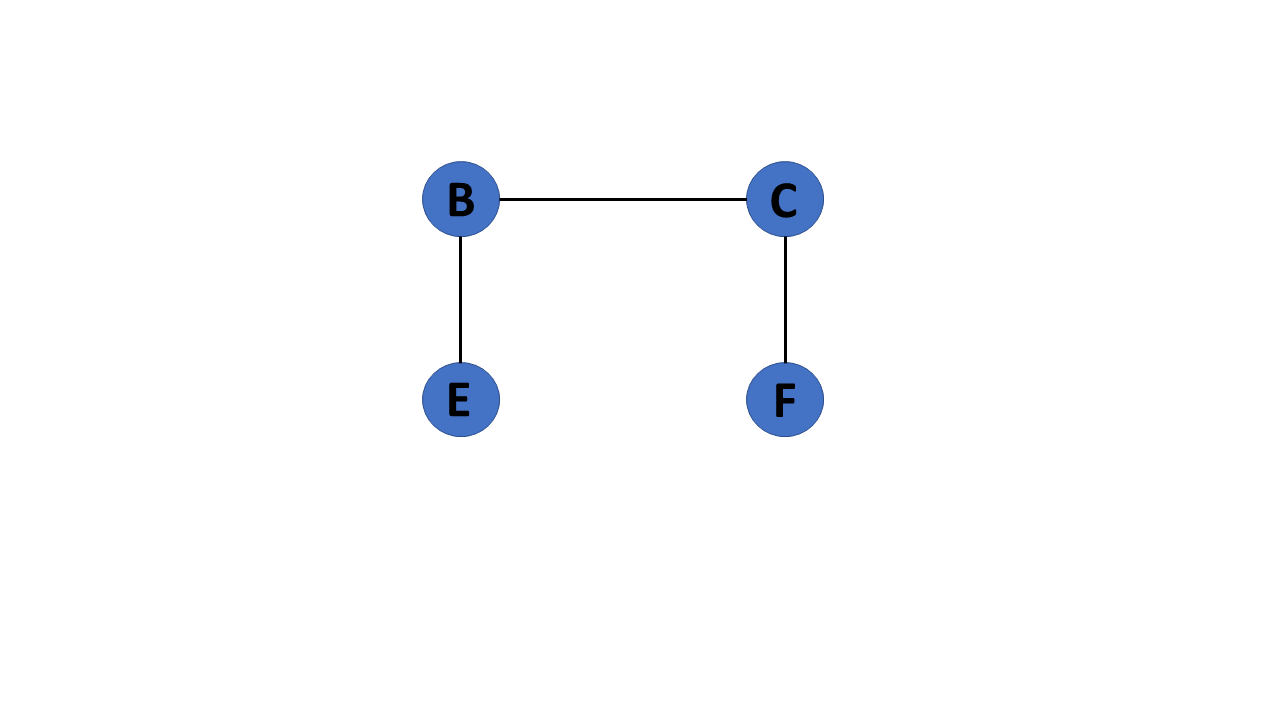### Directed Acyclic Graph

It’s also known as a directed acyclic graph (DAG), and it’s a graph with directed edges but no cycle. It represents the edges using an ordered pair of vertices since it directs the vertices and stores some data.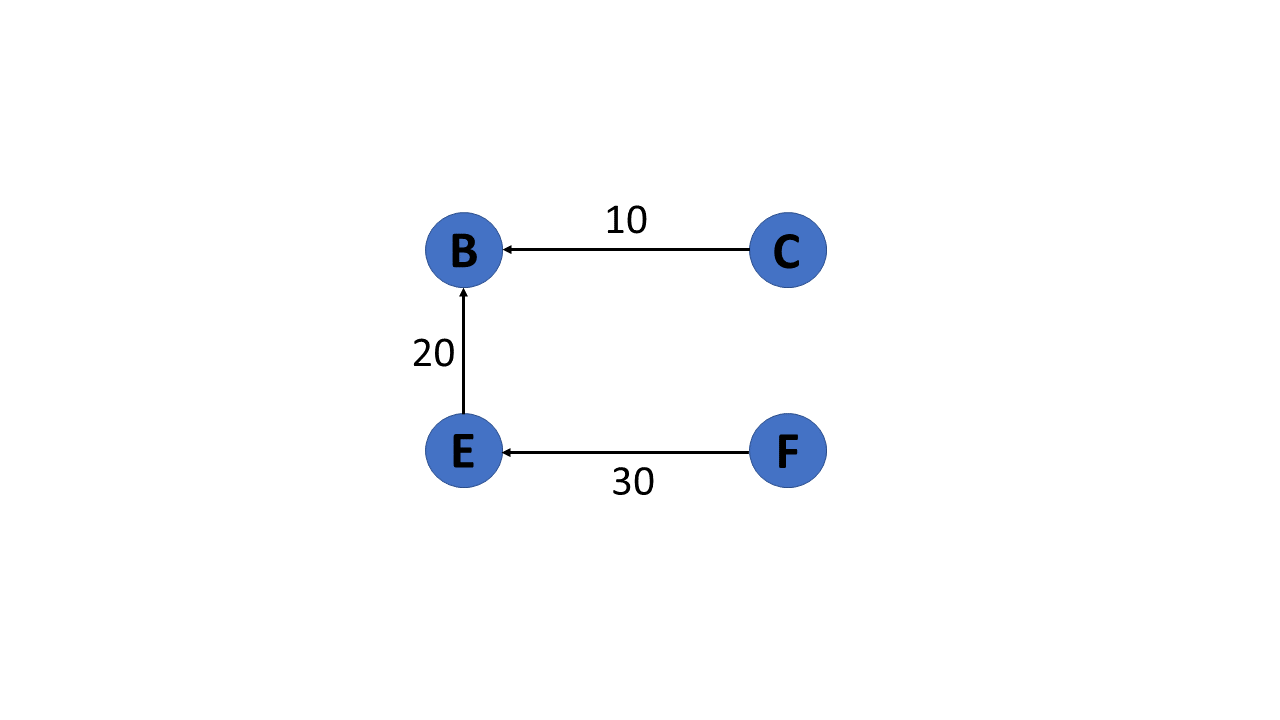### Trees

A tree is a special type of graph that has a root node, and every node in the graph is connected by edges. It’s a directed acyclic graph with a single root node and no cycles. A tree is a special type of graph that has a root node, and every node in the graph is connected by edges. It’s a directed acyclic graph with a single root node and no cycles.### Biprartite Graph

A bipartite graph is a graph whose vertices can be divided into two independent sets, U and V, such that every edge (u, v) either connects a vertex from U to V or a vertex from V to U. In other words, for every edge (u, v), either u belongs to U and v to V, or u belongs to V and v to U. We can also say that there is no edge that connects vertices of same set.#### Examples of Bipartite Graphs

• Authors-to-Papers (they authored)

• Actors-to-Movies (they appeared in)

• Users-to-Movies (they rated)

• Recipes-to-Ingredients (they contain)

• Author collaboration networks

• Movie co-rating networks

#### Projections of Bipartite Graphs### Homogeneous graph

Homogeneous graph consists of one type of nodes and edges, and heterogeneous graph has multiple types of nodes or edges.

An example of a homogeneous graph is an online social network with nodes representing people and edges representing friendship. means we have same node for all types of node and similary all edges are same.

### Heterogeneous graph

Heterogeneous graphs come with different types of information attached to nodes and edges. Thus, a single node or edge feature tensor cannot hold all node or edge features of the whole graph, due to differences in type and dimensionality.

A graph with two or more types of node and/or two or more types of edge is called heterogeneous. An online social network with edges of different types, say ‘friendship’ and ‘co-worker’, between nodes of ‘person’ type is an example of a heterogeneous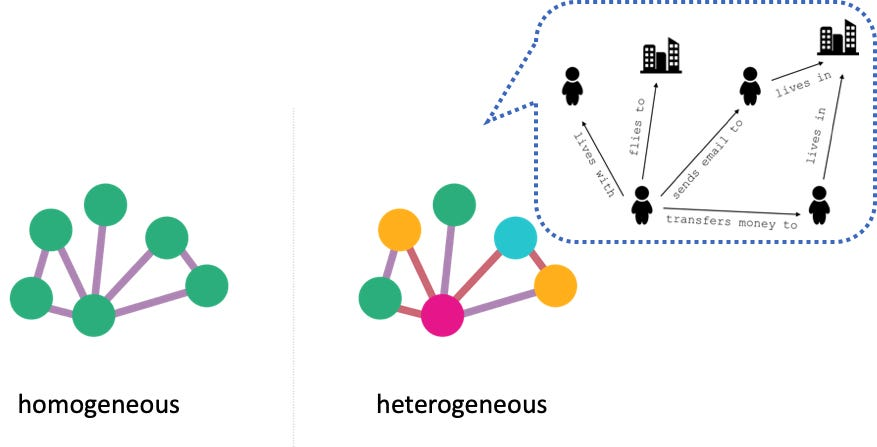## Node degrees

The degree of a node is the number of edges connected to it. A node with no edges is called an isolated node. A node with only one edge is called a leaf node.### Degree of a vertex/Node

The degree of a vertex is the number of edges incident to it. In the following figure, the degree of vertex A is 3, the degree of vertex B is 4, and the degree of vertex C is 2.### In-Degree and Out-Degree of a Vertex/node

In a directed graph, the in-degree of a vertex is the number of edges that are incident to the vertex. The out-degree of a vertex is the number of edges that are incident to the vertex.G = nx.Graph()
G.add_edges_from([('A', 'B'), ('A', 'C'), ('B', 'D'), ('B', 'E'), ('C', 'F'), ('C', 'G')])
print(f"deg(A) = {G.degree['A']}")
DG = nx.DiGraph()
DG.add_edges_from([('A', 'B'), ('A', 'C'), ('B', 'D'), ('B', 'E'), ('C', 'F'), ('C', 'G')])

print(f"deg^-(A) = {DG.in_degree['A']}")
print(f"deg^+(A) = {DG.out_degree['A']}")



Danger

Drawsback of Node Degree feature

• The degree k of node v is the number of edges (neighboring nodes) the node has

• Treats all neighboring nodes equally.

## Node Centrality

Centrality quantifies the importance of a vertex or node in a network. It helps us to identify key nodes in a graph based on their connectivity and influence on the flow of information or interactions within the network.

There are several measures of centrality, each providing a different perspective on the importance of a node:

• Degree centrality

• Engienvector centrality

• Betweenness centrality

• Closeness centrality

Note

• Node degree counts the neighboring nodes without capturing their importance.

• Node centrality $$c_v$$ takes the node importance in a graph into account

## Graph Representation

There are two ways to represent a graph:

2. Edge List

Each data structure has its own advantages and disadvantages that depend on the specific application and requirements.

In an adjacency matrix, each row represents a vertex and each column represents another vertex. If there is an edge between the two vertices, then the corresponding entry in the matrix is 1, otherwise it is 0. The following figure shows an adjacency matrix for a graph with 4 vertices.#### Calculating the degree of node in adjacency matrix

Sum all the 1 in the row corresponding to the node. The sum of the elements in the row corresponding to the node is the degree of the node.1. The adjacency matrix representation of a graph is not suitable for a graph with a large number of vertices. This is because the number of entries in the matrix is proportional to the square of the number of vertices in the graph.

2. The adjacency matrix representation of a graph is not suitable for a graph with parallel edges. This is because the matrix can only store a single value for each pair of vertices.

3. One of the main drawbacks of using an adjacency matrix is its space complexity: as the number of nodes in the graph grows, the space required to store the adjacency matrix increases exponentially. adjacency matrix has a space complexity of $$O\left(|V|^2\right)_{\text {, where }}|V|_{\text{repre- }}$$ sents the number of nodes in the graph.

Overall, while the adjacency matrix is a useful data structure for representing small graphs, it may not be practical for larger ones due to its space complexity. Additionally, the overhead of adding or removing nodes can make it inefficient for dynamically changing graphs.

### Edge list

An edge list is a list of all the edges in a graph. Each edge is represented by a tuple or a pair of vertices. The edge list can also include the weight or cost of each edge. This is the data structure we used to create our graphs with networkx:

edge_list = [(0, 1), (0, 2), (1, 3), (1, 4), (2, 5), (2, 6)]


checking whether two vertices are connected in an edge list requires iterating through the entire list, which can be time-consuming for large graphs with many edges. Therefore, edge lists are more commonly used in applications where space is a concern.

In an adjacency list, each vertex stores a list of adjacent vertices. The following figure shows an adjacency list for a graph with 4 vertices.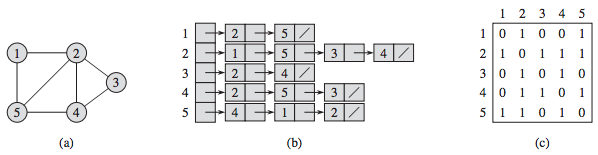However, checking whether two vertices are connected can be slower than with an adjacency matrix. This is because it requires iterating through the adjacency list of one of the vertices, which can be time-consuming for large graphs.

## Graph Traversals

Traversal means to walk along the edges of a graph in specific ways.

Graph algorithms are critical in solving problems related to graphs, such as finding the shortest path between two nodes or detecting cycles. This section will discuss two graph traversal algorithms: BFS and DFS.

Graph traversal is the process of visiting (checking and/or updating) each vertex in a graph, exactly once. Such traversals are classified by the order in which the vertices are visited. The order may be defined by a specific rule, for example, depth-first search and breadth-first search.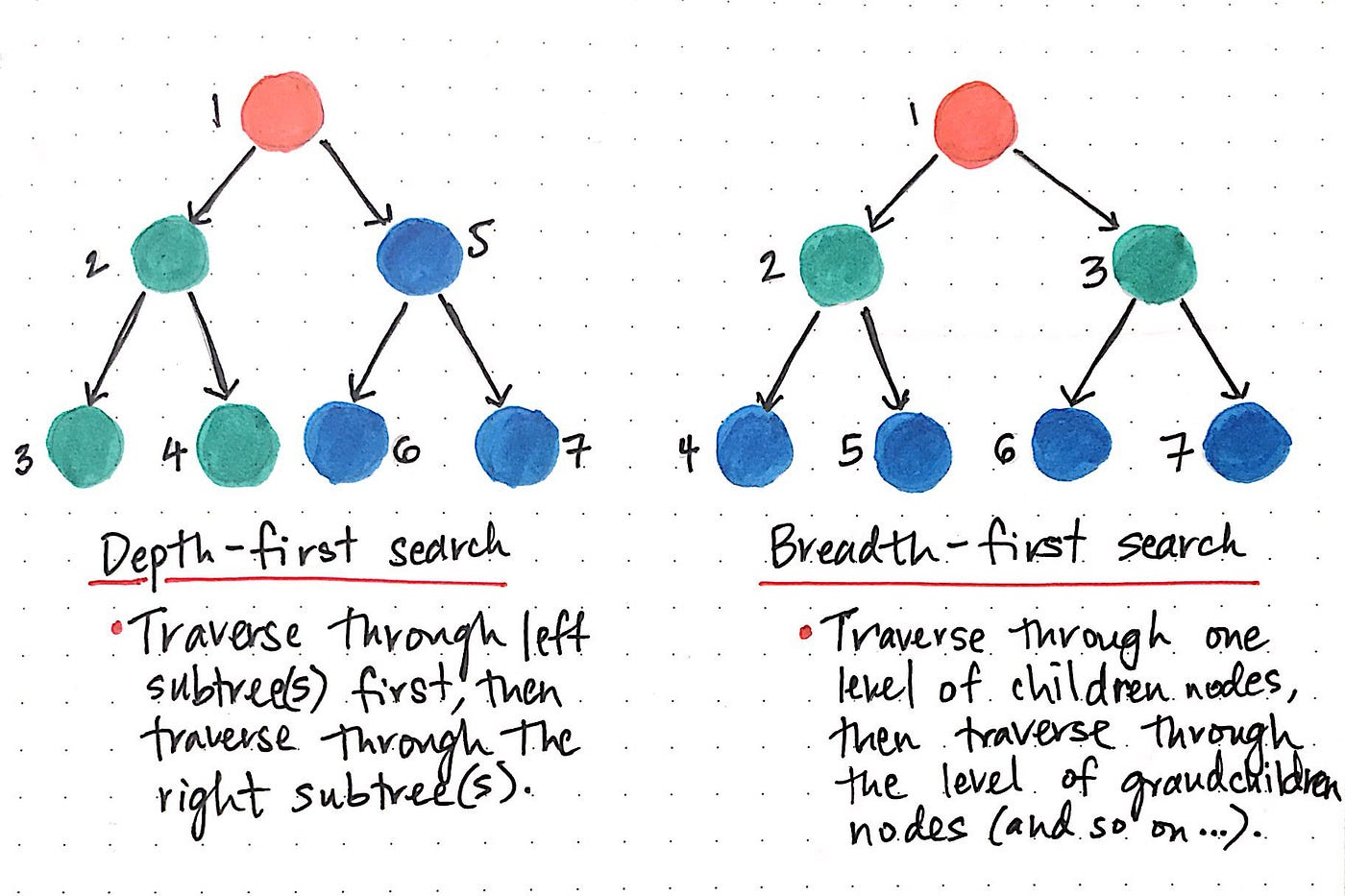While DFS uses a stack data structure, BFS leans on the queue data structure.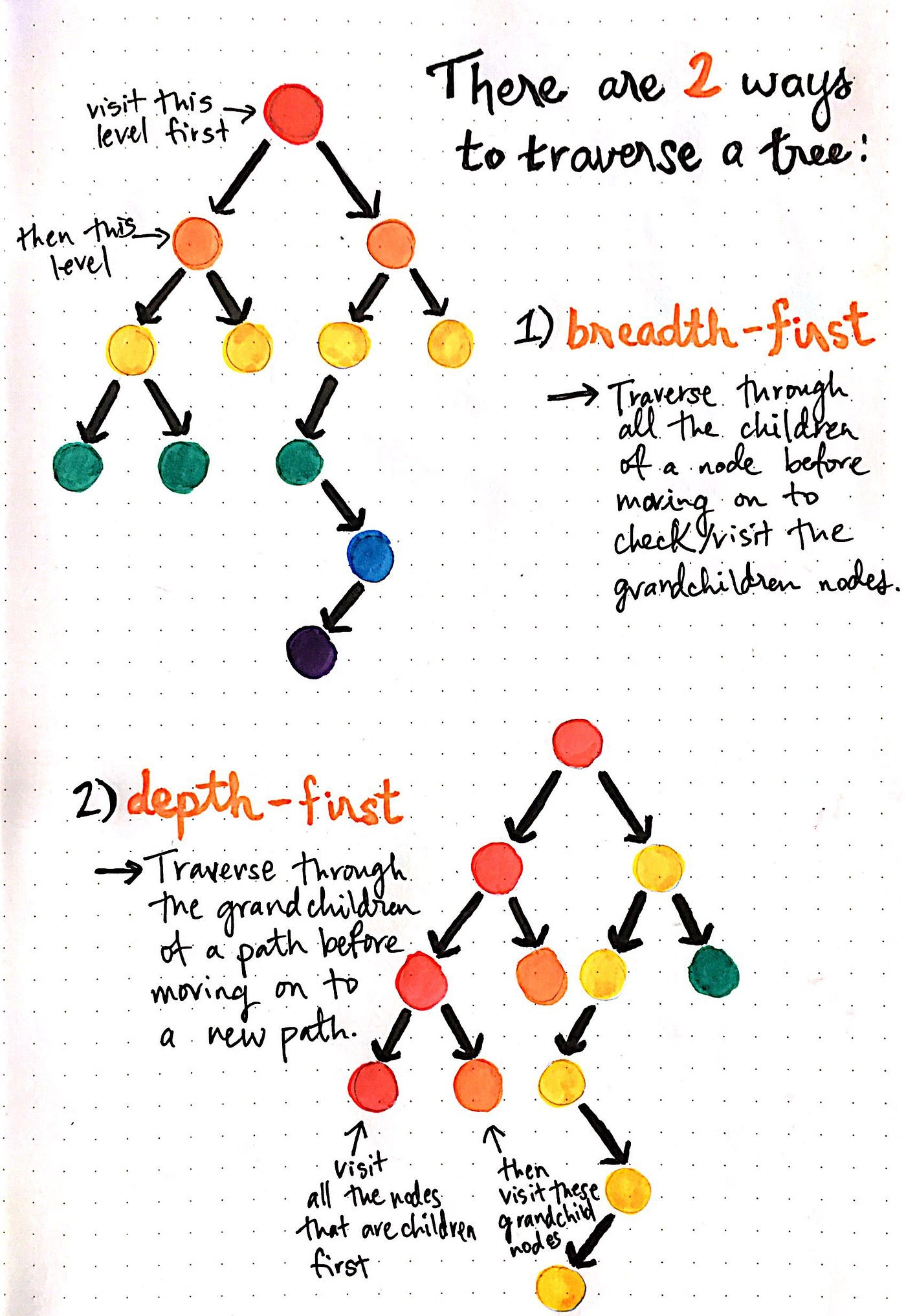### Topological Sort

Topological sorting for Directed Acyclic Graph (DAG) is a linear ordering of vertices such that for every directed edge uv, vertex u comes before v in the ordering. Topological Sorting for a graph is not possible if the graph is not a DAG.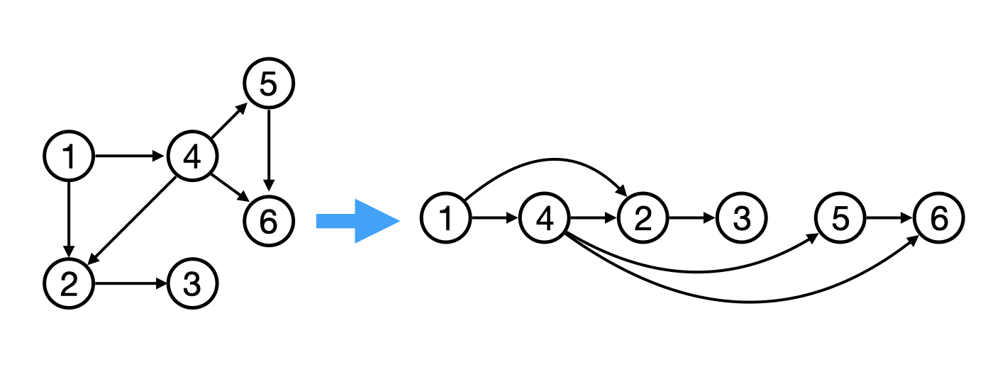### Graph Algorithms

Graph algorithms are used to solve problems that involve graphs. Graph algorithms are used to find the shortest path between two nodes, find the minimum spanning tree, find the strongly connected components, find the shortest path from a single node to all other nodes, find the bridges and articulation points, find the Eulerian path and circuit, find the maximum flow, find the maximum matching, find the biconnected components, find the Hamiltonian path and circuit, find the dominating set, find the shortest path from a single node to all other nodes, find the bridges and articulation points, find the Eulerian path and circuit, find the maximum flow, find the maximum matching, find the biconnected components, find the Hamiltonian path and circuit, find the dominating set, etc.